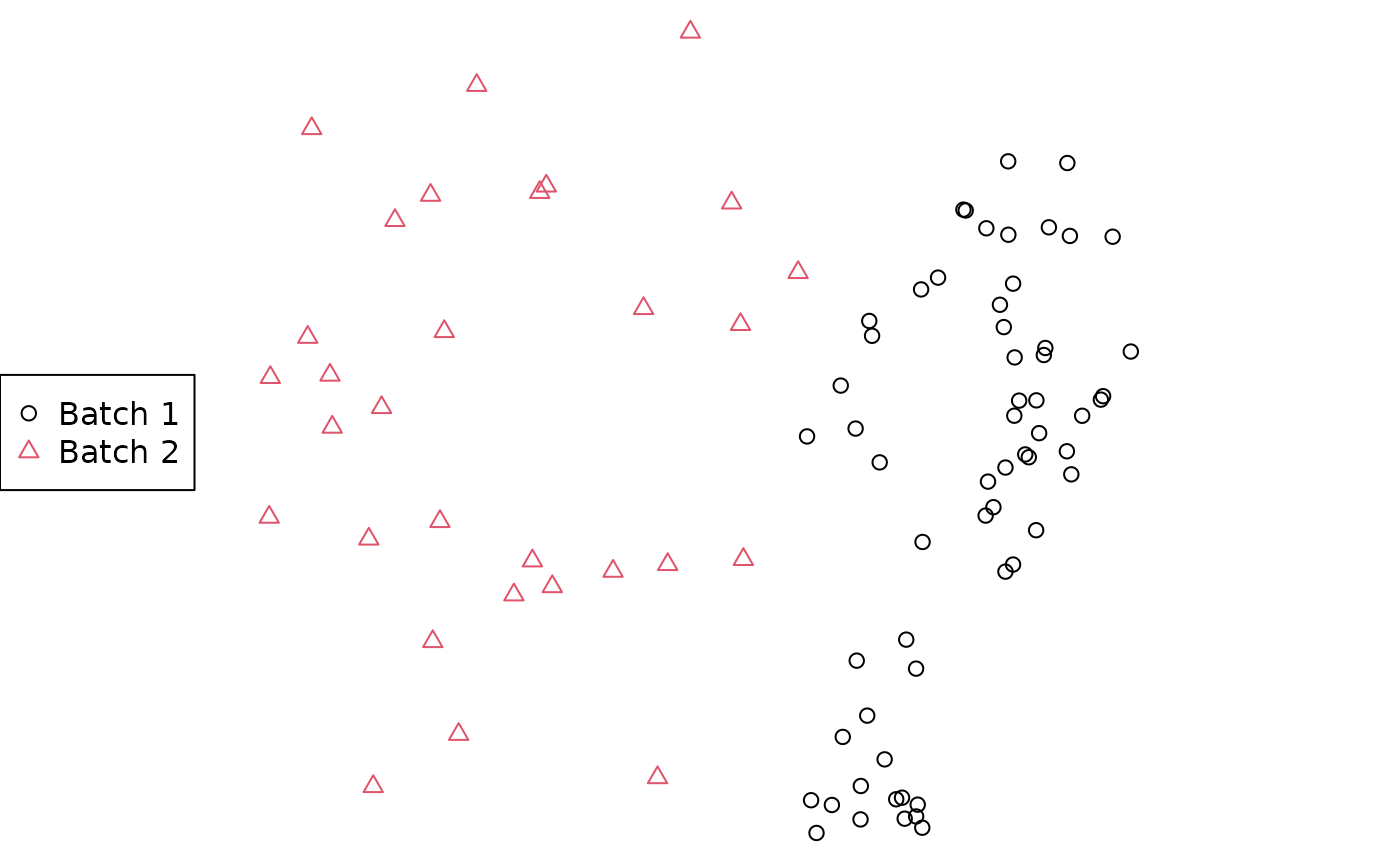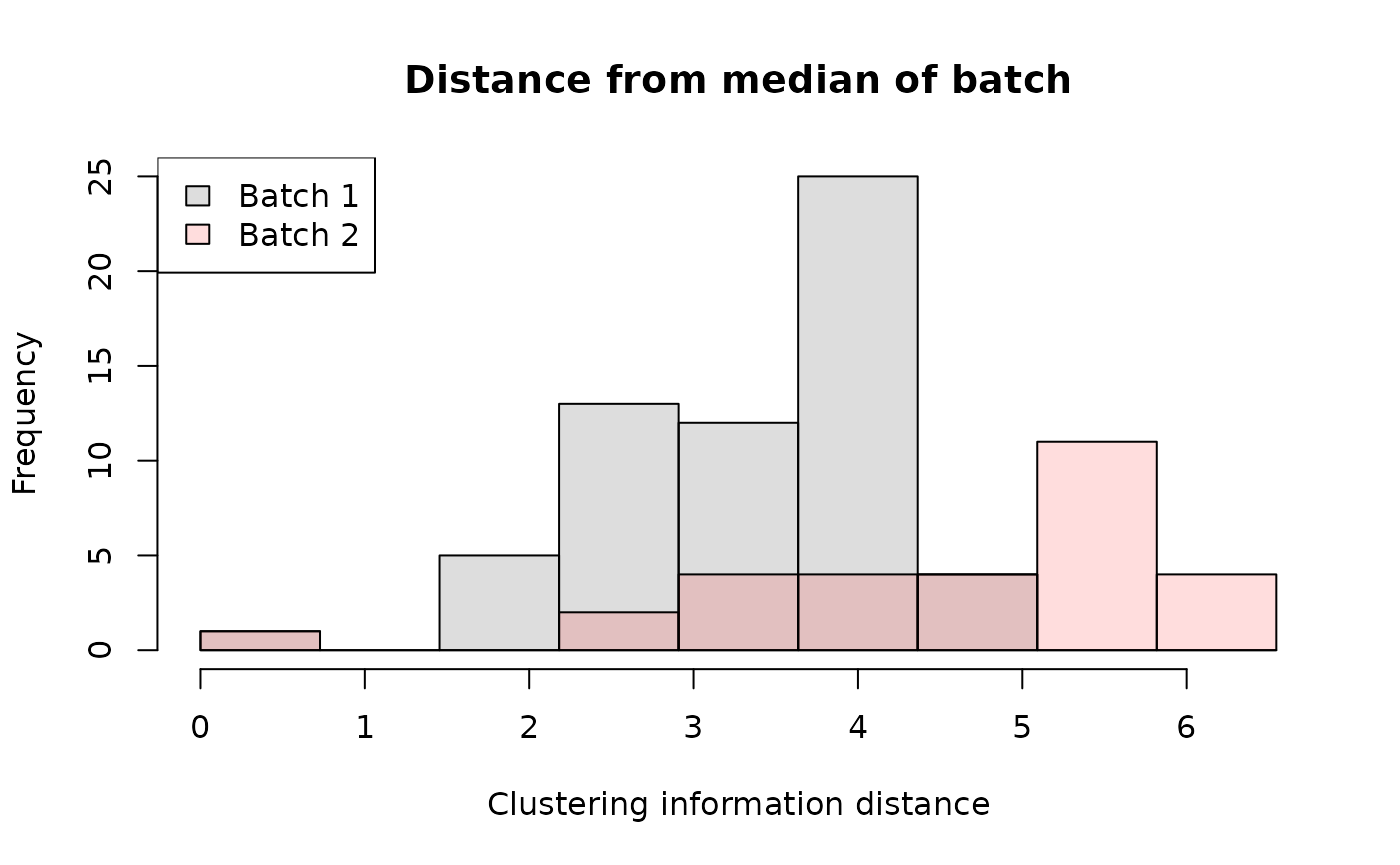A common application of tree space analysis is to compare the outputs of different analyses – for instance, trees obtained from different gene sequences, or results obtained using different models or methods (e.g. Bayesian, maximum likelihood, or parsimony).

## Shiny app

This can be accomplished quickly using the MapTrees() graphical user interface:

• Load trees from file: Select first tree file

• Select an appropriate sample size

• Select Replace existing• On the Display tab, select Point symbols: One per batch, or Colour points by: BatchTree batch styles

## Scripting at the R command line

More control over the mapping can be obtained at the command line:

# Load trees
library("TreeTools", quietly = TRUE)
batch1 <- as.phylo(1:60, 8) # Generate 60 similar trees
batch2 <- as.phylo(seq(200, 800, length.out = 30), 8) # A separate batch of 30 trees
styles <- c(1, 2) # Select plotting colours / symbols
treeStyle <- rep(styles, c(length(batch1), length(batch2)))

# Calculate distances
library("TreeDist")
distances <- ClusteringInfoDistance(c(batch1, batch2))

# Construct over-simple 2D PCoA mapping
mapping <- cmdscale(distances, k = 2)
# Plot mapping
par(mar = rep(0, 4))
plot(mapping,
asp = 1, # Preserve aspect ratio - do not distort distances
ann = FALSE, axes = FALSE, # Don't label axes: dimensions are meaningless
col = treeStyle, # Colour
pch = treeStyle # Plotting symbol
)
legend("left", c("Batch 1", "Batch 2"), col = styles, pch = styles)For more robust analyses than the (potentially misleading!) 2D plot above, consult the companion vignette. Note also that mapped areas and their regions of overlap may not correspond to reality; see the warnings and recommendations in Smith (2022a).

## Comparing trees’ dispersal / hypervolume

Interpreting and comparing the areas of tree space from a projection can be misleading – the expanded apparent area of Greenland under the Mercator projection being a familiar example.Mapping can introduce distortion

As such, it is always best to work with original distances when interpreting whether sets of trees occupy larger or smaller regions of tree space.

### Distances from median

One approach is to plot distances from a median tree:

# Calculate median trees
median1 <- median(batch1)
median2 <- median(batch2)

# Compute distance from each tree to the median of its batch
dist1 <- ClusteringInfoDist(batch1, median1)
dist2 <- ClusteringInfoDist(batch2, median2)

# Set resolution of histogram
nBreaks <- 10
breaks <- seq(0, max(dist1, dist2), length.out = nBreaks)

# Plot first distance set
hist(dist1, col = "#00000022", breaks = breaks,
main = "Distance from median of batch",
xlab = "Clustering information distance",
ylim = c(0, 25) # Omit this line to infer Y axis limit from first batch.
)

hist(dist2, col = "#ff000022", breaks = breaks, add = TRUE)

legend("topleft", c("Batch 1", "Batch 2"),
fill = c("#00000022",  "#ff000022"))In the plotted example, distances to the median tree are greater for batch 2 than batch 1, indicating a more dispersed set of trees that occupies a greater hypervolume. Note that the increased frequency at higher distances is expected: the outer shell of a sphere contains more volume than a layer of equivalent thickness closer to the centre, and this phenomenon becomes more pronounced as the dimensionality of tree space increases.

### Consensus resolution

A complementary approach is to identify the resolution of the consensus of each batch of trees. This approach shares many of the problems with the Robinson–Foulds distance: in particular, resolution can be decimated by a single “rogue” taxon whose position is poorly defined (Smith, 2022b). Detecting and removing rogue taxa can provide a more meaningful point of comparison.

# Create tree set with a rogue taxon

# Set up plotting area
par(mfrow = c(2, 2), mar = rep(0.4, 4))

# Plot naive strict consensus
plot(consensus(batch1, p = 1))
plot(consensus(batch3, p = 1))

if (requireNamespace("Rogue", quietly = TRUE)) {
cons1 <- ConsensusWithout(batch1, p = 1,
Rogue::QuickRogue(batch1, p = 1)[-1, "taxon"])
cons3 <- ConsensusWithout(batch3, p = 1,
Rogue::QuickRogue(batch3, p = 1)[-1, "taxon"])

# The information content of each tree gives a measure of its resolution,
# accounting for omitted rogue leaves
SplitwiseInfo(cons1) # 8.5 bits
SplitwiseInfo(cons3) # 15.1 bits: higher resolution indicates that these
# trees are more similar, notwithstanding rogue taxa.

# Plot the trees
plot(cons1)
plot(cons3)
} else {
message("The package 'Rogue' is required to run this example.")
}Whereas a direct interpretation of this analysis is not straightforward, it can provide a complementary way of understanding the distribution of trees across tree space.

Smith, M. R. (2022a). Robust analysis of phylogenetic tree space. Systematic Biology, syab100. doi: 10.1093/sysbio/syab100

Smith, M. R. (2022b). Using information theory to detect rogue taxa and improve consensus trees. Systematic Biology, syab099. doi: 10.1093/sysbio/syab099